Chapter 8. Electronic Structure of Atoms

# 8.3 Development of Quantum Theory

### Learning Objectives

By the end of this module, you will be able to:

• Extend the concept of wave–particle duality that was observed in electromagnetic radiation to matter as well
• Understand the general idea of the quantum mechanical description of electrons in an atom, and that it uses the notion of three-dimensional wave functions, or orbitals, that define the distribution of probability to find an electron in a particular part of space

The electron shell model gives us a satisfactory picture of the electrons in atom because it accounts for the quantization of the electron energy and it does not constrain the electrons to a fixed orbit.  It also allows us to begin to explain why elements behave the way they do. However many fundamental aspects of the atom are not addressed by this simple model. How exactly do the electrons move? What controls the maximum number of electrons in the shell? And, there are also a number of chemical and physical properties of the elements that remain unexplained by the electron shell model. A more advanced model is required to approach these issues.

## The Wave Mechanics Model of the Atom

Bohr’s ideas were useful but were applied only to the hydrogen atom. However, later researchers generalized Bohr’s ideas into a new theory called quantum mechanics, which explains the behaviour of electrons as if they were acting as a wave, not as particles. Quantum mechanics predicts two major things: quantized energies for electrons of all atoms (not just hydrogen) and an organization of electrons within atoms. Electrons are no longer thought of as being randomly distributed around a nucleus (from Electron Shell Model) or restricted to certain orbits (from Bohr’s Model). Instead, electrons are collected into groups and subgroups that explain much about the chemical behaviour of the atom.

### Wave/Particle Duality and the Uncertainty Principle

We’ve seen that electromagnetic radiation (a form of energy) can be viewed as a wave, or as a particle (a photon, or “packet”). That is, light has a dual nature. If energy can behave like a particle and a wave, can matter also exhibit this duality? It turns out that it does! In the 1920’s Louis Victor de Broglie showed that all moving particles can be described as wave-like. We call this general phenomenon wave/particle duality.

While it may seem odd to think of a physical object, such as a baseball, behaving like a wave, that is just what the theory suggests. However, for any object that is large, the particle nature is dominant and the wave nature is insignificant. But for tiny objects, such as electrons, the wave-like nature is physically meaningful and can be observed.

Around the same time, Werner Heisenberg proposed that the more accurately you know the position of a particle, the less you know about its momentum, and vice versa. This is now called the Heisenberg Uncertainty Principle. This means we can no longer talk about precise trajectories or locations of electrons. Instead, there is always some uncertainty and we can only talk about the PROBABILITY of finding an electron in a certain time and place.

### The Schrödinger Wave Equation and the Wave Mechanics Model

Together, the quantization of energy, wave / particle duality and the Uncertainty Principle all led to Wave Mechanics, developed by Erwin Schrödinger in the 1920’s.

Schrödinger presented his Wave Mechanics in the form of a very complicated mathematical equation which, when solved gives us a series of WAVE FUNCTIONS, each having a specific energy.

A wave function is a mathematical function (like f(x) = x2), and we can plot a graph of the function (Figure 1) to get a picture of the ORBITAL—the space in which the electron is most likely to be found. Depending on the shape of the space, the orbitals have been assigned a name such as: s, p, d, and f. Note that an orbital is much different than an orbit!  An orbit is a set path on which an electron travels. We can’t talk about a set path because of the uncertaintly principle. An orbital is more like a probability map: the region in space where the electron is most likely to be over time.  The differing intensity of the shaded areas is an indication of electron density, which is a measure of the probability of locating an electron in a particular region of space (Figure 1 and 2).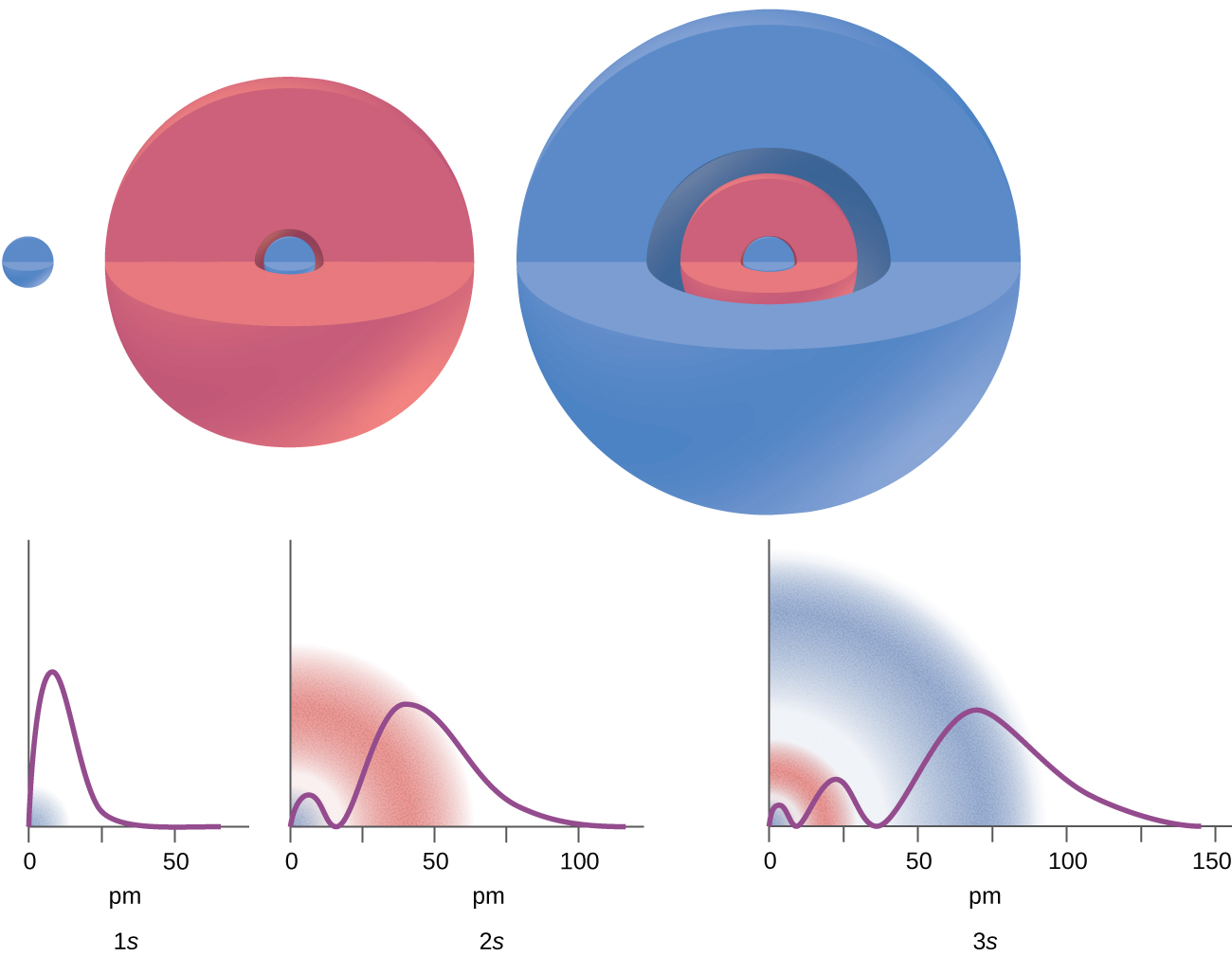Figure 1. This illustrates graphs showing the probability (y axis) of finding an electron for the 1s, 2s, 3s orbitals as a function of distance from the nucleus and how it relates to the space around the nucleus.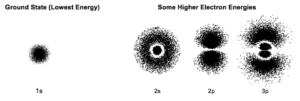Figure 2.  Electron probability maps of lower and higher energy orbitals for the electron in a H atom.

Looking at the probability maps of the electron in hydrogen (Figure 2), we can perhaps best picture an electron as a “cloud”. To simplify the picture of an orbital, however, we generally limit or define the orbital to be the zone within which the electron has 90% probability of being contained, and we represent this space with a solid. Figure 3 shows the many different complex shapes that the electron movement traces out. The s subshell electron density distribution is spherical and the p subshell has a dumbbell shape. The d and f orbitals are more complex. These shapes represent the three-dimensional regions within which the electron is likely to be found.  Keep in mind the “cloud” image when viewing these representations however.

## Describing atoms using the Wave Mechanics Model

### Properties of electrons in orbitals

Orbitals are labeled with a number (known as the “shell”) followed by a letter (s, p, d, or f, known as the “subshell”), for example 1s, 2s, 2p, 3s. The subshells occur with a certain multiplicity; there are always seven f orbitals, five d orbitals, three p orbitals, and one s orbital (these could be called the “sub-subshells”). Any atom has all of these orbitals though they may or may not be occupied by electrons; those orbitals that are not occupied are available for possible excited states. Each orbital has a relative energy as shown in Figure 4, where the orbitals are represented as lines.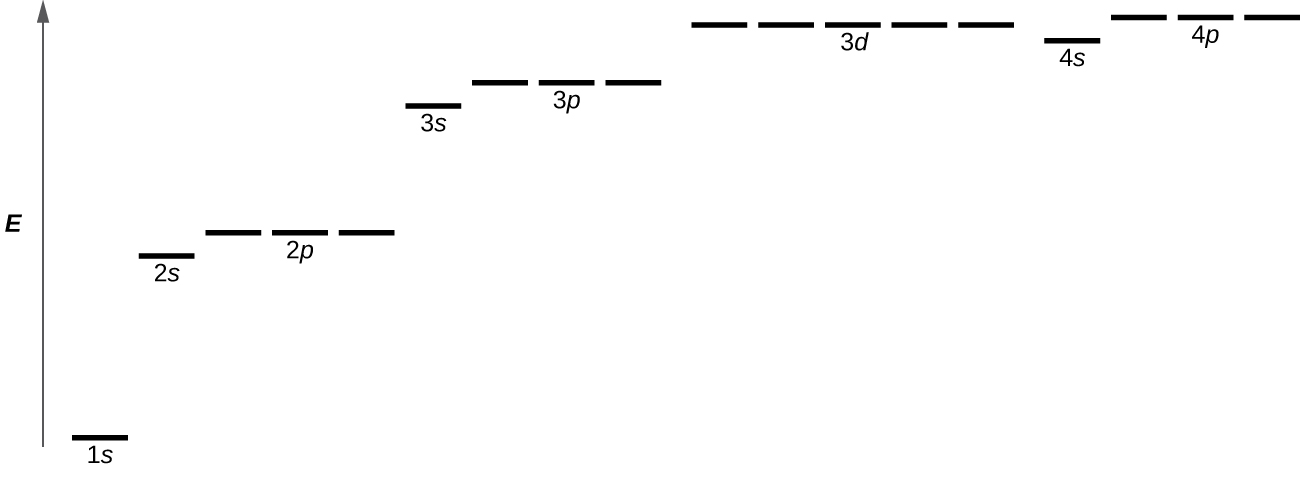Figure 4. The chart shows the energies of electron orbitals in a multi-electron atom (up to 4p).

Electrons and other subatomic particles behave as if they are spinning (we cannot tell if they really are, but they behave as if they are). Electrons themselves have two possible spin states, spin up (graphically represented as an upward pointing arrow, ˦ ), and spin down (graphically represented as a downward pointing arrow, ˨ ). An orbital can hold a maximum of two electrons; when an orbital has two electrons in it, one electron will be spin up, and one will be spin down. This last aspect is an outcome of the Pauli Exclusion Principle, which states that no two electrons in the same atom can behave identically.

## Key Concepts and Summary

The quantum mechanical model of atoms describes the three-dimensional position of the electron in a probabilistic manner. Therefore, atomic orbitals describe the areas in an atom where electrons are most likely to be found.

### Exercises

1. How are the Bohr model and the quantum mechanical model of the hydrogen atom similar? How are they different?

2. Draw out the 1s, 2s and 3s orbitals and describe the differences and similarities between them.

3. Draw out the 2px, 2py and 2pz orbitals and describe the differences and similarities between them.

4. Draw out the 3p and 3s orbitals and describe the differences and similarities between them.

5. The following represents examples of what type of orbital?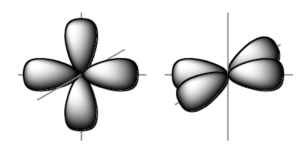1. Both models have a central positively charged nucleus with electrons moving about the nucleus in accordance with the Coulomb electrostatic potential. The Bohr model assumes that the electrons move in circular orbits that have quantized energies, angular momentum, and radii that are specified by a single quantum number, n = 1, 2, 3, …, but this quantization is an ad hoc assumption made by Bohr to incorporate quantization into an essentially classical mechanics description of the atom. Bohr also assumed that electrons orbiting the nucleus normally do not emit or absorb electromagnetic radiation, but do so when the electron switches to a different orbit. In the quantum mechanical model, the electrons do not move in precise orbits (such orbits violate the Heisenberg uncertainty principle) and, instead, a probabilistic interpretation of the electron’s position at any given instant is used, with a mathematical function ψ called a wavefunction that can be used to determine the electron’s spatial probability distribution. These wavefunctions, or orbitals, are three-dimensional stationary waves that can be specified by three quantum numbers that arise naturally from their underlying mathematics (no ad hoc assumptions required): the principal quantum number, n (the same one used by Bohr), which specifies shells such that orbitals having the same n all have the same energy and approximately the same spatial extent; the angular momentum quantum number l, which is a measure of the orbital’s angular momentum and corresponds to the orbitals’ general shapes, as well as specifying subshells such that orbitals having the same l (and n) all have the same energy; and the orientation quantum number m, which is a measure of the z component of the angular momentum and corresponds to the orientations of the orbitals. The Bohr model gives the same expression for the energy as the quantum mechanical expression and, hence, both properly account for hydrogen’s discrete spectrum (an example of getting the right answers for the wrong reasons, something that many chemistry students can sympathize with), but gives the wrong expression for the angular momentum (Bohr orbits necessarily all have non-zero angular momentum, but some quantum orbitals [s orbitals] can have zero angular momentum).

2. Differences: n values are different therefore the energies of the orbitals are different (1s is lower in energy, 2s higher in energy and 3s is highest in energy) and size of the orbitals are different (1s smaller, 2s bigger, 3s biggest).

Similarities: they are all s-orbitals, therefore their shape are the same (spherical), they can only contain two electrons with opposite spins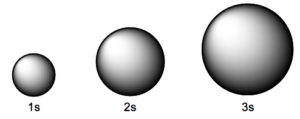3. Differences: the orientation of the orbitals is different

Similarities: n values are the same therefore their energies of the orbitals and size of the orbitals are the same; they are all p-orbitals, therefore their shape are the same (dumbbell); they can only contain two electrons with opposite spins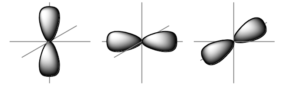4. Differences: one is a p-orbital and the other is an s-orbital, therefore their shapes are different (dumbbell and spherical), p-orbitals can have different orientations, but s-orbitals don’t

Similarities: n values are the same therefore their energies of the orbitals and size of the orbitals are the same; they can only contain two electrons with opposite spins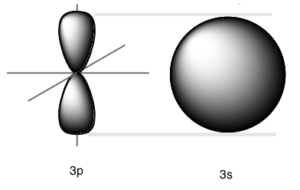5. These are two examples of d-orbitals.

## Glossary

atomic orbital: mathematical function that describes the behavior of an electron in an atom (also called the wavefunction), it can be used to find the probability of locating an electron in a specific region around the nucleus, as well as other dynamical variables

d orbital: region of space with high electron density that is either four lobed or contains a dumbbell and torus shape. An electron in this orbital is called a d electron

electron density: a measure of the probability of locating an electron in a particular region of space, it is equal to the squared absolute value of the wave function ψ

f orbital: multi-lobed region of space with high electron density. An electron in this orbital is called an f electron

Heisenberg uncertainty principle: rule stating that it is impossible to exactly determine both certain conjugate dynamical properties such as the momentum and the position of a particle at the same time. The uncertainty principle is a consequence of quantum particles exhibiting wave–particle duality

p orbital: dumbbell-shaped region of space with high electron density. An electron in this orbital is called a p electron

Pauli exclusion principle: specifies that no two electrons in an atom can have the same value for all four quantum numbers

quantum mechanics: field of study that includes quantization of energy, wave-particle duality, and the Heisenberg uncertainty principle to describe matter

s orbital: spherical region of space with high electron density. An electron in this orbital is called an s electron

wavefunction (ψ): mathematical description of an atomic orbital that describes the shape of the orbital; it can be used to calculate the probability of finding the electron at any given location in the orbital, as well as dynamical variables such as the energy and the angular momentum